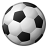# How to Set up an expression in arcpy to calculate percent where one of the variables is the result of a previous calculation

2857
20
08-13-2020 10:49 AMNew Contributor III

Hi,

I have a feature class with two fields I am interested in using.  One field is "area_acres" and the other is "Survey_Pcnt".  I have calculated the field "area_acres".  My intent is to sum all the values in the field "area_acres" and then use that "summed_total" in the following expression to calculate the "Survey_Pcnt" field:  "!area_acres!/summed_total*100".  I have managed to sum all the values successfully, but when I try to add summed_total as a variable in the expression and run the script I get the error: TypeError: unsupported operand type(s) for /: 'str' and 'float'.  This error leads me to believe I'm trying to perform division between a string and floating point which wouldn't work.  Below is my code that I'm having trouble with.

summed_total = sum((r for r in arcpy.da.SearchCursor(env.workspace + "/APE_intersect_dissolve_adequacy", ['area_acres'])))
print (summed_total)

percentage_expression = "(float(!area_acres!))"/float(summed_total)*"(float(100))"

I have tried writing the percentage_expression many different ways to no avail.  At this point any help is greatly appreciated. Let me know if there is any other information I can provide.  I am using ArcGIS desktop 10.7.1 and arcpy.

thank you,

John

Tags (2)
1 Solution

Accepted SolutionsbyMVP Esteemed Contributor

maybe you have to emulate a codeblock and expression

``````exp = "do_stuff(!area_acres!, summed_total)"
codeblock = """
def do_stuff(area, tot):
return area/tot * 100"""‍‍‍‍``````

... sort of retired...
20 RepliesbyEsri Community Moderator

Hi John.  This would be a good question for the Python‌ space!New Contributor III

Thank you Kory.  Can you tell me how to get it there?

thanks,

JohnNew Contributor III

Looks like you moved it for me, thank you!byEsri Community Moderator

No worries.  Yeah, I figured the @mention would make it show up in that space so hopefully somebody there can help!byMVP Esteemed Contributor

e = float(!area_acres!)/float(summed_total) * float(100)

Will return a correct numeric value, now if the expression needs to be quoted, add the quotes to this.  You had too many

... sort of retired...New Contributor III

Hi Dan,

thank you for your reply unfortunately e = float(!area_acres!)/float(summed_total) * float(100) gives me a syntax error which I'm trying to resolve.  When I put double quotations around the whole expression, the variables become strings and the float command does not work.  I'll keep trying and responses are still welcome.

thank you,

JohnbyMVP Esteemed Contributor

maybe you have to emulate a codeblock and expression

``````exp = "do_stuff(!area_acres!, summed_total)"
codeblock = """
def do_stuff(area, tot):
return area/tot * 100"""‍‍‍‍``````

... sort of retired...New Contributor III

Hi Dan,

That worked with the condition of adding a new field of the total area and calculating it first.  So all fields need to be created and populated before I can use the above code block.  I was interested in a method of using the sum of the areas without having to create a new field and populate it with area sums (that are the same value).  Otherwise it worked.

thank you,

JohnNew Contributor

I have a similar question and I tried to do the same as yours but my code is not working...

```ExecuteError: ERROR 000539: Traceback (most recent call last):
File "<expression>", line 1, in <module>
NameError: name 'summed_total' is not defined

Failed to execute (CalculateField).```

-summed_total = sum((r for r in arcpy.da.SearchCursor("summary_tract_2", ['Sum_tract'])))
print(summed_total)

per_exp = "my_percent(!Sum_tract!, summed_total)"

codeblock = """
def my_percent(area, tot):
return area/tot * 100"""

print(codeblock)